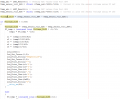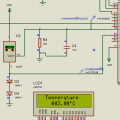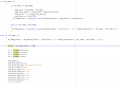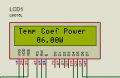# proteus simulation not producing expected output from code

#### onejcb

Joined Sep 26, 2012
2
Hi all I hope someone is able to help.

Please see code images attached below.

Here is a brief explanation of how it is expected to work. Ignoring the error due to resolution at the moment, using proteus AN4 and AN5 values the code should calculate something like.

Voltage_Diff = (Temp_sensor_volt_AN4 - Temp_sensor_volt_AN5);
Voltage_Diff = 403.632 - 372.436 = 31.196 "please note proteus values are multiplied by 1000 in code where Vref 5000 instead of 5V)

else
{
PV_temp = (unsigned long)((Voltage_Diff)/10);
PV_temp = 31.196/10 = 3.1196

STC_Temp = 20 "declared in a header file"
Temp_Coefficient = 0.0039 "declared in a header file"

if (PV_temp > 0) "in this case PV_temp is greater than 0"
{
if (PV_temp <= STC_Temp) "in this case PV_temp is 3.1196 or 3.00 when resolution is factored."
{
temp_diff = STC_Temp - PV_temp; "in this case temp_diff = 20 - 3 = 17"
temp_coef_factor = temp_diff * Temp_Coefficient; "in this case temp_coef_factor = 17 * 0.0039 = 0.0663"
temp_whole = 1 + temp_coef_factor; "in this case temp_whole = 1+0.0663 =1.0663"
PV_Temp_Power = (unsigned long)(PV_Design_Power * temp_whole * irad_factor); "in this case PV_Temp_Power = 20 * 1.0663 * 1 = 21.326"
}

ptemp1 = PV_Temp_Power * 100; "in this case ptemp1 = 21.326 * 100 = 2132.6

c1 = (ptemp1/1000)%10;
c2 = (ptemp1/100)%10;
c3 = (ptemp1/10)%10;
c4 = (ptemp1/1)%10;

Lcd_Clear();
Lcd_Set_Cursor(1,1);
Lcd_Print_String("Temp Coef Power");
Lcd_Set_Cursor(2,5);
Lcd_Print_Char(c1+'0'); "in this case this should print out 2"
Lcd_Print_Char(c2+'0'); "in this case this should print out 1"
Lcd_Print_String("."); "in this case this should print out ."
Lcd_Print_Char(c3+'0'); "in this case this should print out 3"
Lcd_Print_Char(c4+'0'); "in this case this should print out 2"
Lcd_Print_String("W"); "in this case this should print out W"
__delay_ms(80);

however output I am getting is 06.00W instead of 21.32W printed on LCD screen as shown in attached image below.